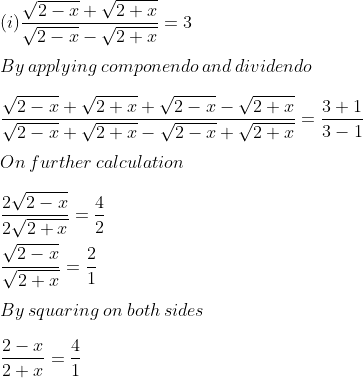Guru

# Find x from the following equations : (i){Root(2−x) +root(2+x)}/{root (2−x) −root (2+x)} =3

• 0

exam oriented an important question from ML aggarwal, class 10th, chapter 7, ratio and proportion, Avichal publication

In this question we have been given a equation with variable x and we have to find the value of x

Question 11, 7.3

Share

1. Solution:By cross multiplication

8 + 4x = 2 – x

So we get

4x + x = 2 – 8

5x = – 6

x = -6/5

• 0